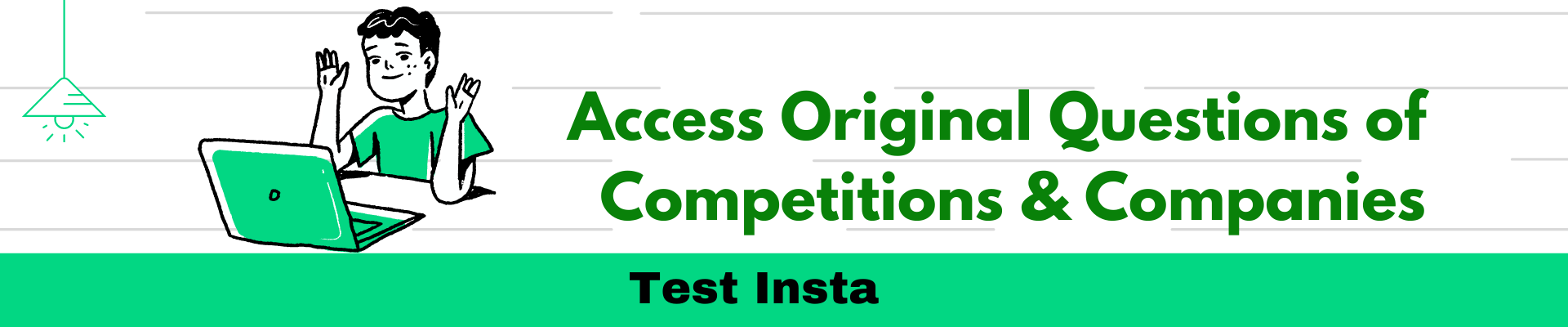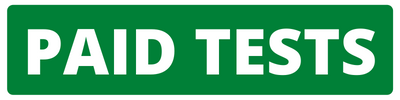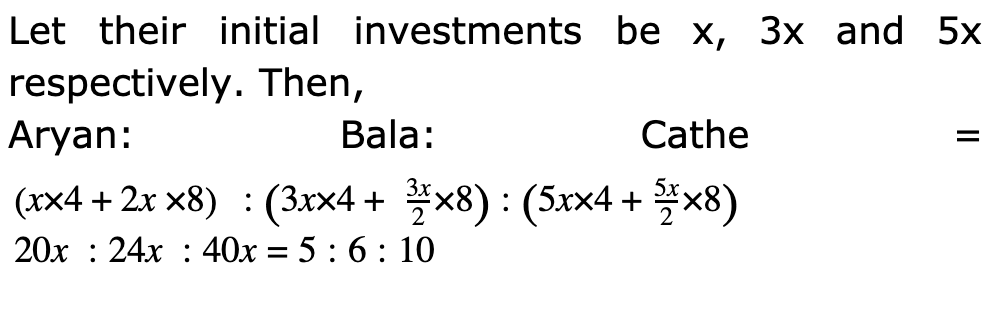CAT | Accenture Quants Questions

## Accenture Quants QuestionsJobs By Batch
Jobs By Location
Jobs By Degree
Jobs By Branch
IT Jobs
Internships
Govt. Jobs• ##### Original Questions of IT Companies| All Topics### Quiz Begins Here

Q #1
:

#### Quants Question

Directions: Read the following information to answer the question.

Aryan, Bala, Cathe started a business with their investments in the ratio 1:3 :5. After 4 months, A invested the same amount as before and B as well as C withdrew half of their investments. The ratio of their profits at the end of the year is :
+Q #3
:

#### Quants Question

Directions: Read the following information to answer the question.

A reduction of 20% in the price of sugar enables a 1 purchaser to obtain 2 1/2 kg more for Rs 160. Find the original price per kg of sugar.
+

Reduction on Rs 160 = 20    × 160 = Rs 32 100

Reduced price of 2 1/2    kg of sugar = 32

Reduced price of 1 kg of sugar = 32 × 5

If reduced price is Rs 80, then original = Rs 100

If reduced price is Rs    64/5 , then original =    100/80 ×64/5        = Rs 16

Q #4
:

#### Quants Question

Directions: Read the following information to answer the question.

Reema took a loan of Rs 1200 with simple interest for as many years as the rate of interest. If she paid Rs. 432 as interest at the end of the loan period, what was the rate of interest.
+

Let rate = R% then Time = R years.

=>1200∗R∗R100=432=>R2=36=>R=6%